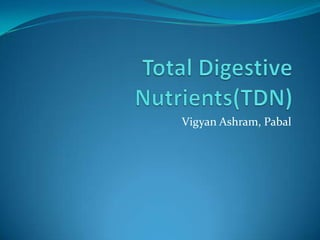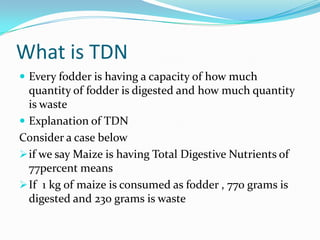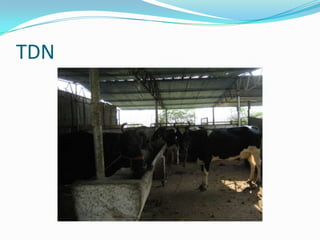Se está descargando tu SlideShare. ×

# Total digestive nutrients(TDN)

Anuncio
Anuncio
Anuncio
Anuncio
Anuncio
Anuncio
Anuncio
Anuncio
Anuncio
Anuncio
AnuncioCargando en…3
×

1 de 16 Anuncio

# Total digestive nutrients(TDN)

This slideshow explains about what is TDN Total Digestive Nutrients for fodder management of dairy animals

This slideshow explains about what is TDN Total Digestive Nutrients for fodder management of dairy animals

Anuncio
Anuncio

### Total digestive nutrients(TDN)

1. 1. Vigyan Ashram, Pabal
2. 2. What is TDN  Every fodder is having a capacity of how much quantity of fodder is digested and how much quantity is waste  Explanation of TDN Consider a case below if we say Maize is having Total Digestive Nutrients of 77percent means If 1 kg of maize is consumed as fodder , 770 grams is digested and 230 grams is waste
3. 3. TDN
4. 4. What is the use of finding TDN  A question obviously comes in mind what is the use of finding TDN  By finding TDN , a farmer can calculate exact quantity of how much fodder to be given to cattle  This is useful in dairy and goat farming
5. 5. Required quantities for calculating TDN Quantities Required for TDN calculation How much percent TDN the fodder type has How much is the weight of animal How much TDN should be given per day to cattle
6. 6. How much TDN fodder types have  Following is the table of fodder type and its TDN Content  They are already calculated and used as standard Reference
7. 7. TDN Chart
8. 8. Weight of animal to be calculated  Weight of animal calculation is given in a earlier PowerPoint presentation  Video Link for finding cow weight  Please click here for a video in Marathi for taking measurement of cattle weight 
9. 9. How much TDN required for Cattle  From the previous slides, we have came across following things  TDN Content in fodder  Weight calculation of animal Now since we know weight, here is one standard of how much TDN is to be given daily The above calculation is a standard one Total Amount of TDN to be given daily is 10 grams per kg of weight of cattle
10. 10. Calculation of fodder requirements of cattle based on TDN  Consider a case given below  Cattle weight is 500 kgs.  Fodder to be given is combination of jowar stems( ) ,green maize, groundnut cake(Total 3 fodder types) Task to Do  We have to calculate daily fodder requirements for one cattle
11. 11. Calculation  As we have seen earlier  Weight of cow = 500 kg  Hence TDN Required = 500 x 10 = 5000 gms = 5 kg  Now we have 3 fodder types as seen earlier  Total TDN to be given = TDN Required / fodder types  Total TDN = 5000 / 3 = 1667 grams for each fodder  ie 1667 grams of green maize,jowar stems and groundnut cake each Standard Amount of TDN to be given daily is 10 grams per kg of weight of cattle
12. 12. Formula Fodder Weight = 100 x TDN to be given Standard Available TDN
13. 13. Applying the formula  By applying the formula, we will calculate how much green maize we will require for fulfilling the TDN  Available TDN for green maize is 17%(Refer chart earlier)  Which means that for 1667 gms of TDN, we will require ??? kg of green maize  Applying the formula, we get  Fodder weight = 100 x 1667/17 = 9805 grams  Thus we will require 9.805 kgs of green maize daily for a cow of 500 kgs
14. 14. Calculating other types of fodder requirements  We have seen earlier for green maize  Now we will calculate for other remaining fodder items For Jowar stems  Fodder weight = 100 x 1667 / 50(Refer the TDN chart) We require Jowar stems = 3334 grams = 3.334 kgs / day For Groundnut cake  Fodder weight = 100 x 1667 / 71(Refer TDN Chart)  We require 2.347 kgs of Groundnut cake /day
15. 15. Final calculations  Thus we have seen that for a 500 kg weight cow, To maintain the TDN we require fodder as shown below Fodder Requirements per day for a 500 kg weight cow 9.805 grams of green maize 3.34 kgs of jowar stems 2.347 kgs of groundnut cake Total of 15.492 kgs / approx 15.5 kgs of fodder per day for 1 cow of 500 kgs
16. 16. Assignment  Referring to earlier slide  Calculate cost per kg for the fodder items  Make total sum of cost of fodder items per day  Prepare a monthly budget for a cow for fodder